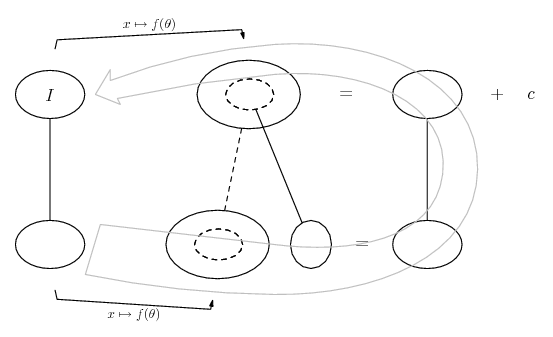# Thread: Integration by parts

1. ## Integration by parts

Hi all, I need some help. I'm taking system dynamics and control over the summer, but it's been over 3 years since my last calculus course, and some of these integrals are killing me.

$\displaystyle \int_1^{2.5} \frac{1}{0.05-0.02\sqrt{H}}\,dH$

is evaluated to be

$\displaystyle -250ln(0.05-0.02\sqrt{H})-100\sqrt{H}+250$ before plugging integration limits, but I can't even get here.

I've tried substituting the lower half with X, then going from there which gets me a partial answer, and I've tried integrating by parts. I'm so out of mental shape it hurts.

Happy 4th

2. ## Re: Integration by parts

Parts will get you there. How did you do it?

You may wish to simplify your life a little, unless you are married to significant figures, and rewrite as $\displaystyle \frac{100}{5-2\sqrt{H}}$.

3. ## Re: Integration by parts

$\displaystyle u = \frac {100} {5-2\sqrt{H}}$
$\displaystyle dv = dH \, v = H$
$\displaystyle du = 100 ln(5-2\Sqrt{H})\frac {-2}{\sqrt{H}}\,dH$

$\displaystyle \frac {100H} {5-2\sqrt{H}} + 200 \int_1^{2.5} \sqrt{H}ln(5-2\sqrt{H})\, dH$

Have I made a mistake anywhere? If no, how do I go about evaluating the vdu integral?

4. ## Re: Integration by parts

Well done. You might have resisted trying to simplify $\displaystyle \frac{H}{\sqrt{H}}$. You'll need that back.Try a substitution: $\displaystyle u = 5 - 2\sqrt{H}$.

5. ## Re: Integration by parts

$\displaystyle u = 5 - 2\sqrt{H}$
$\displaystyle du = \frac{-2}{\sqrt{H}}\,dH$
$\displaystyle \frac{100H}{5-2\sqrt{H}} + 200 \int_1^{2.5} H ln(u) du$

where does the leftover H term go? do you just consider it another constant now? and how do I have to alter the limits of integration for this again?

I would really appreciate it if you could just give me a step by step. This would be like a quick review, then. I took calc 1 in 2004, I haven't been in school for 2.5 years, and I'm jumping back to finish my last year playing catchup in higher engineering classes, and I learn best by seeing a solution then picking it apart.

6. ## Re: Integration by parts

Use your definition of 'u' and solve for H. Good work. That's kind of a mess. Of course, there's still some more work to go.

Note: There may be other (and possibly MUCH EASIER) ways.

7. ## Re: Integration by parts

I don't think parts is working for you there - getting your u's and du's muddled. I would of course recommend lazy integration by parts...

http://www.ballooncalculus.org/examp...nce.html#parts

... but not in this case. A simple u-sub would be H = u^2.

Just in case a picture helps...i.e. replace H with something... as we said, u^2...... where (key in spoiler) ...

Spoiler:... is the chain rule. Straight continuous lines differentiate downwards (integrate up) with respect to the main variable (in this case u), and the straight dashed line similarly but with respect to the dashed balloon expression (the inner function of the composite which is subject to the chain rule).

The general drift is...So,

$\displaystyle F(u^2) = \sqrt{u^2} + \frac{5}{2} \ln |5 - 2\sqrt{u^2}| + c$

i.e...

$\displaystyle I = \sqrt{H} + \frac{5}{2} \ln |5 - 2\sqrt{H}| + c$

_________________________________________

Don't integrate - balloontegrate!

Balloon Calculus; standard integrals, derivatives and methods

Balloon Calculus Drawing with LaTeX and Asymptote!

#### Search Tags

integration, parts